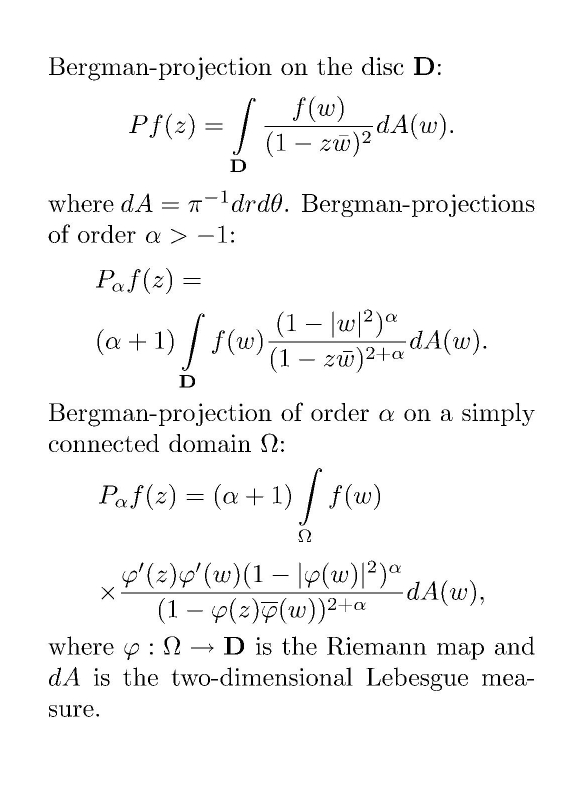Main page Department of Mathematics and Statistics of University of HelsinkiFormula 1. Bergman projections.Formula 2. A new reprocuding formula. Let Ω be a complex domain (in one or, respectively, N ≥ 2 dimensions). Bergman space Ap = Ap(Ω) with 1 ≤ p < ∞ is the closed subspace of Lp consisting of analytic functions. The Lp -space is defined with the normalized 2-dimensional, respectively, 2N-dimensional, Lebesgue measure dA. Bergman-projection is the orthogonal projection from L2 onto A2. In the case of the unit disc or a simply connected domain in one dimension there is an integral formula for the projection, see Formula 1. If the Lp -norm is endowed with a weight which is the αth power of the boundary distance, one can still define the Bergman projection of order α analogously. In the unweighted case, the Bergman projection is not bounded with respect to the sup norm (p= ∞). The situation is different in the weighted cases like in Formula 2. Papers mentioned on this page contain studies related to weighted sup-norms. SOME RECENT ARTICLES P.Erkkilä, J.Taskinen: Sup-norm estimates for Bergman projections on regulated domains. Math.Scand. 102, 1 (2008), 111-130. This work is an adoptation of the paper in Ann.Acad.Sci.Fenn (2003) to the case p = ∞. We consider simply connnected regulated domains and the general Bergman projections (see Formula 1) on them. We give sufficient and necessary conditions for their boundedness in terms of the largest or smallest angle in the boundary curve of the domain Ω. See the citation for more details on regulated domains. J.Bonet, M.Engliš, J.Taskinen: Weighted L∞-estimates for Bergman projections. Studia Math.171,1 (2005), 67-92. This paper contains three subtopics. First, we prove a new reproducing formula (see Formula 2); it contains a general analytic function which comes from a systematic developement of the idea of forming analytic functions of polynomials of order α appearing in projections in Formula 1. We show that the projection with exponential kernel (see Formula 2) is bounded simultaneuosly for all weighted spaces with arbitrary β. Second, we give a partial generalization to p = ∞ of Bekolle's Studia Math. (1982) paper, where he gives Muckenhoupt-type conditions for weights, characterizing boundedness of Bergman projections on weighted Lp-spaces. The third part contains a related consideration to solve positively the topological subspace problem for weighted inductive limits. The weight families under consideration satisfy logarithmic growth conditions. M.Engliš, T.Hänninen, J.Taskinen: Minimal L∞-type spaces on strictly pseudoconvex domains on which the Bergman projection is continuous. Houston J. Math. 32,1 (2006). This is a nontrivial generalization of the paper below to the setting of strictly pseudoconvex domains in higher dimensions. J.Taskinen: On the continuity of the Bergman and Szegö projections. Houston J. Math. 30,1 (2004), 171-190. Motivated by the problem that the Bergman projection is not bounded with the unweighted sup-norm, we introduce the spaces H, analytic, and L, measurable, functions on the disc, which grow at the boundary at most like a power of logarithm of the boundary distance. The spaces are inductive limits, and H is a closed subspace of L. Both of them are algebras (this property is missed by the hyperbolic BMO space and the Bloch space). Finally, the Bergman projection is a continuous operator from L onto H. The corresponding space of harmonic functions and the Szegö projection also fit into this theory in the natural way. Back to main page .